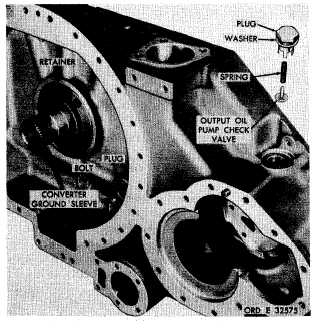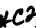D E S C R I P T I ON
Custom Search*C2 PAR  137-141 T O R Q U E     C O N V E R T E R     H O U S I N G     R E B U I LD C H A P   5,   SEC   X V S e c t i o n    X V .    T O R Q U E    C O N V E R T E R    H O U S I N G — R E B U I L D 1 3 7 .    D E S C R I P T I ON Refer   to   par.   9   for   the   description   of   the torque    converter    housing. 1 3 8 .    D I S A S S E M B L Y    ( f i g .    3 7 4 ,    f o l d - o u t    3 ) .   U s i n g     a     1 - l / 2 - i n c h     w r e n c h ,     r e m o ve o u t p u t    o i l    p u m p    c h e c k    v a l v e    p l u g ,    w a s h e r, valve   and   spring   (fig.   156). .   U s i n g    a    9 / 1 6 - i n c h    w r e n c h ,    r e m o v e five   self-locking   bolts   retaining   seal   ring   re- t a i n e r    ( f i g .    1 5 6 ) .    R e m o v e    t h e    r e t a i n e r. .   D o    n o t    r e m o v e    t h e    t o r q u e    c o n v e r t er g r o u n d    s l e e v e    u n l e s s    r e p l a c e m e n t    i s    n e c e s- sary   (fig.   156).   If   necessary,   press   the   sleeve from   the   housing   toward   the   front   side. .   R e m o v e    a n y    p l u g s    i n    t h e    h o u s i n g    if necessary   to   aid   in   the   cleaning   of   oil   passages. N o t e .    I f    n e c e s s a r y    t o    r e m o v e    i n s e r ts or   dowels,   refer   to   par.   72   for   inspec- tion    and    repair    recommendations. 1 3 9 .     C L E A N I NG Refer   to   par.   71   for   cleaning   recommen- d a t i o n s. 1 4 0 .    I N S P E C T I O N    A N D    R E P A IR R e f e r    t o    p a r .    7 2    f o r    g e n e r a l    i n s p e c t i on a n d    r e p a i r    r e c o m m e n d a t i o n s .    R e p a i r    a n d    r e - b u i l d    p o i n t s    o f    m e a s u r e m e n t    f o r    f i t s ,    c l e a r - ances   and   wear   limits   are   indicated   by   small, lower case letters in fig. 374, fold-out 3. Refer   to   par.   237   for   wear   limits   information. Figure  156.  Torque  converter  housing  and  related  parts 1 4 1 .    A S S E M B L Y    ( f i g .    3 7 4 ,    f o l d - o u t    3) a.  Install  any  plugs  in  housing  which  were removed   to   aid   in   the   cleaning   of   the   torque converter   housing   (fig.   156). .   I f    t h e    c o n v e r t e r    g r o u n d    s l e e v e    w as r e m o v e d ,    i n s t a l l    a    n e w    r e p l a c e m e n t    g r o u n d s l e e v e    ( f i g .    1 5 6 ) .    P r e s s    s l e e v e    i n t o    h o u s i ng until   firmly   seated,   being   sure   that   the   bolt holes   in   the   housing   and   sleeve   are   properly indexed. .   Install   the   seal   ring   retainer   and   secure with   five   3/8-16   self-locking   bolts   (fig.   156). Torque   the   bolts   to   36-43   pound-feet. .   Install   output   oil   pump   valve,   spring, washer   and   plug   (fig.   156). 1 21Integrated Publishing, Inc. - A (SDVOSB) Service Disabled Veteran Owned Small Business